Asymmetric Mask Layer Generation in OptoDesigner

# Asymmetric Mask Layer Generation in OptoDesigner

In OptoDesigner, the concept of a Mask Cross-Section (MCS) was introduced to abstract away the physical implementation of a waveguide from the design intent. The user draws what they would like to get on the chip, and the MCS concept makes sure that all the correct mask layers are generated, all following the exact shapes that the user specified. This feature highlight shows exactly how that is to be implemented by a PDK developer and shows the full range of capabilities.

A simple example is show below, where the design intent is a simple 90-degree bend, but the mask layers required for that are trenches around the waveguide – essentially, a local inverse of the design intent.Figure 1. Trench layer generation from design intent. Left: intent; RIght: trench layers.

The example above is typically implemented by associating the MCS with two virtual mask layers, a ‘core’ that follows the design intent directly, and a ‘cladding’ that is a widened version of the intent. During the export of the mask, the ‘core’ is then subtracted from the ‘cladding’ layer to derive the final mask layers that will be used to etch the trenches. The script lines that associate two such layers with an MCS would be: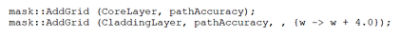The arguments to the mask::AddGrid function are the mask layer, the discretization accuracy (which controls the maximum distance between the analytical curve and the mask polygon), and a function that relates the width of a layer to the width of the design intent. In this example, the ‘cladding’ layer follows the design intent at 2 micrometer distance on both sides. This could be any function; if you know your process has a width-dependent bias (which would mean that the deviation between the actual width on the chip and the width on the mask depends on the width), you can directly compensate for this in the width function.

In addition to width-dependent mask layers, it is also possible to directly define asymmetric mask layers. This allows for a different way to define the trenches above, which no longer needs any Boolean operations among virtual mask layers: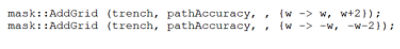Such asymmetric definitions require a function returning two numbers. These numbers are the locations (measured perpendicularly from the heart line of the design intent curve) where the mask layer is to be turned on and off. Note that ‘w’ is half the width here, while in the symmetric case w is the full width.

One can go further with this concept; why only attach a single layer? Using asymmetric mask layers, it is even possible to create all the layers needed for a PN junction silicon photonics modulator. In the simplified version we show here, the ‘trench’ layer defined a shallow etch into the silicon; the purple and orange (non-filled) layers are p and n doping in the silicon, meeting in the middle to create a junction. A contact layer (dark green) creates an electrical connection between the doped silicon and the (yellow) metal layer that is used for connectivity. By attaching all these layers to a single MCS by means of the script below, a single curve definition by the user automatically creates all required layers (Figure 2):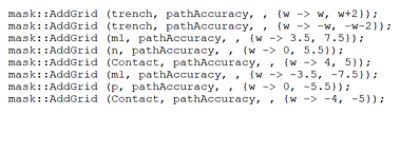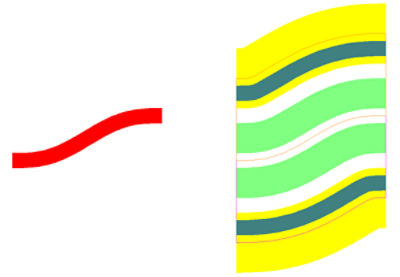Figure 2. Layer generation for a PN junction waveguide geometry. Left: design intent; Right: all mask layers.

If one does not make the mask open-close locations depend on w, the masks will still follow the heart line, but will be unaffected by the width. In the example below, only the inner edge of the trenches follows the waveguide width, the others just follow the heart line: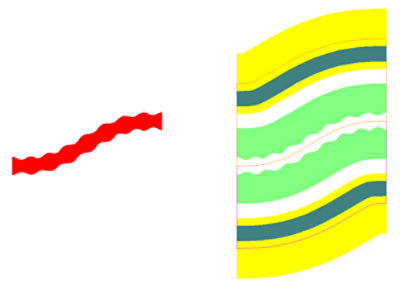Figure 3. Layer generation for grating in a PN junction waveguide geometry. Left: design intent; Right: all mask layers.